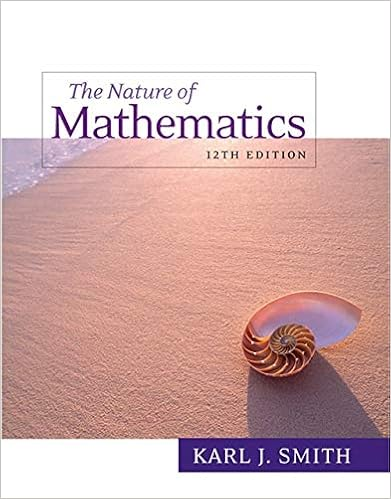# Math191_Week11_section - Math 191 1 Evaluate the following...

• Notes
• 1

This preview shows page 1 out of 1 page.

##### We have textbook solutions for you!
The document you are viewing contains questions related to this textbook.The document you are viewing contains questions related to this textbook.
Chapter 18 / Exercise 17
Nature of Mathematics
SmithExpert Verified
Math 191 Problem Set for Week 10 Section 1. Evaluate the following integrals using integration by parts: a. R cos - 1 ( x 2 ) dx b. R e - 2 x sin 3 xdx 2. Evaluate the integrals. It may be necessary to use a substitution first.
3. Evaluate the integrals (1) without using a trigonometric substitution, (2) using a trigonometric substi- tution.
##### We have textbook solutions for you!
The document you are viewing contains questions related to this textbook.The document you are viewing contains questions related to this textbook.
Chapter 18 / Exercise 17
Nature of Mathematics
SmithExpert Verified
•••